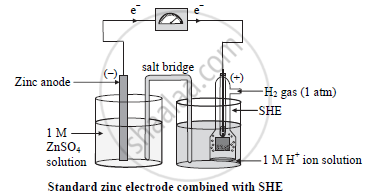# Construct a Labelled Diagram for the Following Cell - Chemistry

Diagram

Construct a labelled diagram for the following cell:

Zn|Zn^(2+)(1M)||H^+(1M)|H_(2(g,1atm))|Pt

#### Solution

In the given representation of the cell, a standard Zn2+(1M)|Zn electrode is combined with standard hydrogen electrode to form the cell:

Zn|Zn^(2+)(1M)||H^+(1M)|H_(2(g,1atm))|PtConcept: Electrochemical Cells
Is there an error in this question or solution?
2016-2017 (July)

Share